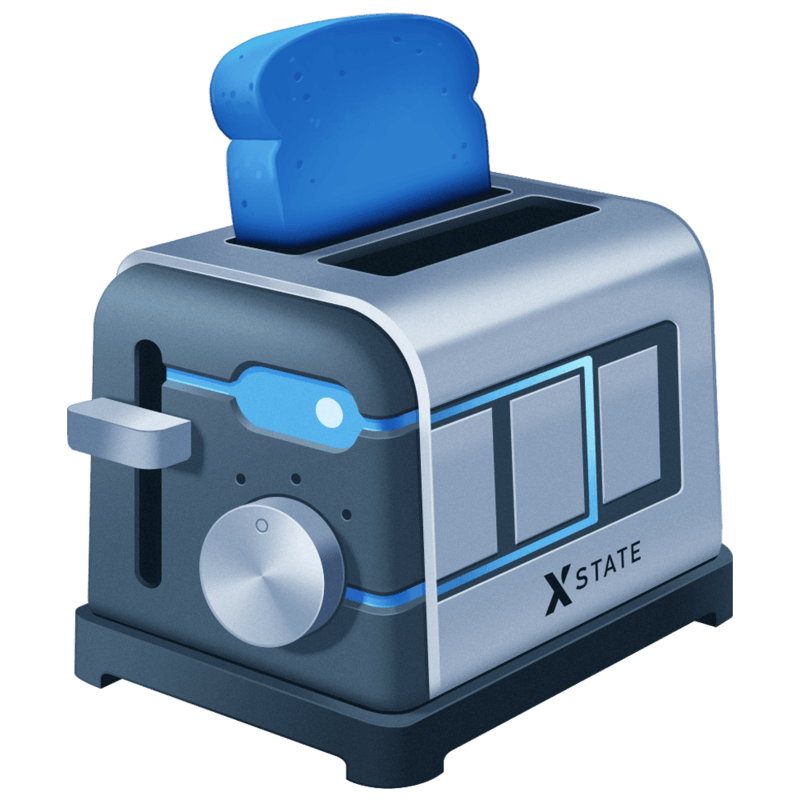## clamp()

``const clamp = (min, max) => number => Math.min(Math.max(min, number), max)``

I have found this function useful again and again in my career, often serving as a way to prevent broken number values from getting somewhere without being to obtrusive to the user.

It can take a moment to understand how `clamp` works because it's a bit counterintuitive. We make use of `Math.min` and `Math.max` to accomplish `clamp`.

`Math.min` will return you the minimal value of a set of numbers.

``console.log(Math.min(76, 24, -42)) // -42``

`Math.max` will return to you the maximal value of a set of numbers.

``console.log(Math.max(76, 24, -42)) // 76``

Therefore, we can create a `clamp` by composing these together. First we make sure that the number is not below our `min` with:

``const minThresholdResult = Math.max(min, number)``

You can hopefully see that if `number` is less than `min`, `Math.max` will return `min`, therefore clamping the result to the `min` value. We pass this result into our next check, ensuring the value is not above `max` with:

``const result = Math.min(minThresholdResult, max)``

If the value of `minThresholdResult` is higher than our `max` value, it will clamp it to the `max` value of our range, otherwise it will return our value.

Here it is in use:

``````const between0and100 = clamp(0, 100)

const numbers = [42, 142, -42]

numbers.forEach(number => {
console.log(between0and100(number))
})

// 42
// 100
// 0``````

### Let's talk some more about JavaScript, React, and software engineering.

I write a newsletter to share my thoughts and the projects I'm working on. I would love for you to join the conversation. You can unsubscribe at any time.Introduction to State Machines and XState

## Check out my courses!

Liked the post? You might like my video courses, too. Click the button to view this course or go to Courses for more information.
View on egghead.io